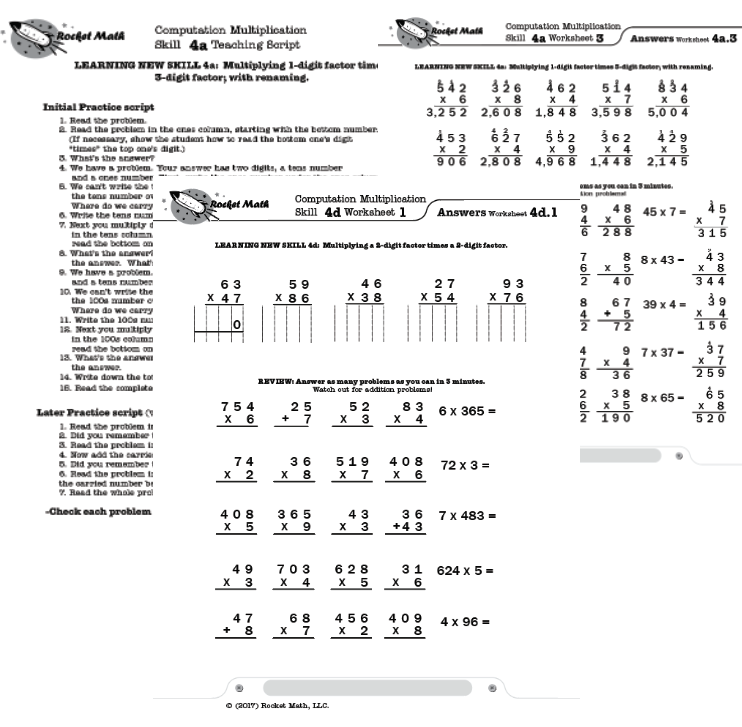## Teach how to do (compute) multi-step multiplication problems

After becoming fluent with multiplication facts, the best way for students to retain the knowledge of those facts is by doing multiplication computation.  If students have not been taught multiplication computation, this program breaks it down into small, easy-to-learn steps that are numbered in a teaching sequence that leaves nothing to chance.

## Assess to find where to begin instruction

Included in the Learning Track is an assessment to help you find out where to start instruction in the sequence. Test the student and begin teaching with the first skill on which they have difficulty.

## Skills taught in this Learning Track

Note that the number for each skill gives the grade level as well as indicating the teaching sequence.  Skill 3b is a 3rd grade skill and after skill 3e is learned the next in the sequence is skill 4a.  The sequence of skills is drawn from M. Stein, D. Kinder, J. Silbert, and D. W. Carnine, (2006) Designing Effective Mathematics Instruction: A Direct Instruction Approach (4th Edition) Pearson Education: Columbus, OH.

(3b) Multiplying 1-digit times 2-digit; no renaming

(3c) Multiplying 1-digit times 2-digit; carrying

(3d) Multiplying 1-digit times 2-digit, written horizontally.

(3e) Reading and writing thousands numbers, using commas.

(4a) Multiplying 1-digit times 3-digit

(4b) Multiplying 1-digit times 3-digit; zero in tens column

(4c) Multiplying 1 digit times 3 digit, written horizontally

(4d) Multiplying 2-digits times 2-digits.

(4e) Multiplying 2-digits times 3-digits.

(5a) Multiplying 3-digits times 3-digits.

(5b) Multiplying 3-digits times 3-digits; zero in tens column of multiplier.

## How to teach this Learning Track

For each skill there is a suggested Teaching Script giving the teacher/tutor/parent consistent (across all the skills we use the same explanation) language of instruction on how to do the skill.  The script helps walk the student through the computation process.  For the teacher, in addition to the script, there are answer keys for the five worksheets provided for each skill.

Each worksheet is composed of two parts.  The top has examples of the skill being learned that can be worked by following the script.  After working through those examples with the teacher the student is then asked to work some review problems of addition problems that are already known.  The student is asked to do as many as possible in 3 minutes—a kind of sprint.  If all is well the student should be able to do all the problems or nearly all of them, but finishing is not required.  Three minutes of review is sufficient for one day.

There are five worksheets for each skill.  Gradually as the student learns the skill the teacher/tutor/parent can provide progressively less help and the student should be able to do the problems without any guidance by the end of the five worksheets.  There are suggestions for how to give less help in the teaching scripts.